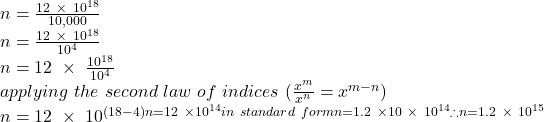## Intel estimates that about 12 quintillion transistors are shipped around the globe each year. If that represents 10,000 times the number of

Question

Intel estimates that about 12 quintillion transistors are shipped around the globe each year. If that represents 10,000 times the number of ants on the planet, find the number of ants on Earth. (Show all your workings. Final answer MUST standard notation.)

in progress 0
6 months 2021-08-13T02:07:21+00:00 1 Answers 0 views 0

Number on ants on Earth = 1.2 × 10¹⁵

Step-by-step explanation:

1 quintillion = 10¹⁸ or (1000000000000000000)

∴ 12 quintillion = 12 × 10¹⁸

12 quintillion = 10,000 × Number of ants on Earth

Let the number of ants on Earth = n

12 quintillion = 10,000 × n

dividing both sides by 10,000

12 quintillion ÷ 10,000 = nNumber on ants on Earth = 1.2 × 10¹⁵